# How to count cells with text in Excel: any, specific, filtered cells

How do I count cells with text in Excel? There are a few different formulas to count cells that contain any text, specific characters or only filtered cells. All the formulas work in Excel 365, 2021, 2019, 2016, 2013 and 2010.

Initially, Excel spreadsheets were designed to work with numbers. But these days we often use them to store and manipulate text too. Want to know how many cells with text there are in your worksheet? Microsoft Excel has several functions for this. Which one should you use? Well, it depends on the situation. In this tutorial, you will find a variety of formulas and when each formula is best to be used.

## How to count number of cells with text in Excel

There are two basic formulas to find how many cells in a given range contain any text string or character.

### COUNTIF formula to count all cells with text

When you wish to find the number of cells with text in Excel, the COUNTIF function with an asterisk in the criteria argument is the best and easiest solution:

COUNTIF(range, "*")

Because the asterisk (*) is a wildcard that matches any sequence of characters, the formula counts all cells that contain any text.

### SUMPRODUCT formula to count cells with any text

Another way to get the number of cells containing text is to combine the SUMPRODUCT and ISTEXT functions:

SUMPRODUCT(--ISTEXT(range))

Or

SUMPRODUCT(ISTEXT(range)*1)

The ISTEXT function checks if each cell in the specified range contains any text characters and returns an array of TRUE (cells with text) and FALSE (other cells) values. The double unary (--) or the multiplication operation coerces TRUE and FALSE into 1 and 0, respectively, producing an array of ones and zeros. The SUMPRODUCT function sums all the elements of the array and returns the number of 1's, which is the number of cells that contain text.

To gain more understanding of how these formulas work, please see which values are counted and which are not:

 What is counted What is not counted Cells with any text Special characters Numbers formatted as text Visually blank cells that contain an empty string (""), apostrophe ('), space or non-printing characters Numbers Dates Logical values of TRUE and FALSE Errors Blank cells

For example, to count cells with text in the range A2:A10, excluding numbers, dates, logical values, errors and blank cells, use one of these formulas:

`=COUNTIF(A2:A10, "*")`

`=SUMPRODUCT(--ISTEXT(A2:A10))`

`=SUMPRODUCT(ISTEXT(A2:A10)*1)`

The screenshot below shows the result: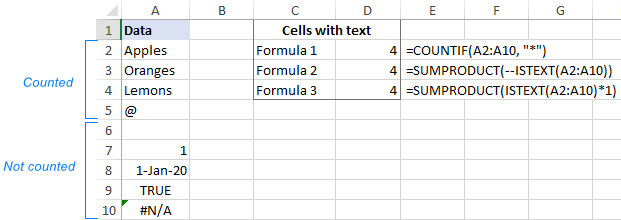## Count cells with text excluding spaces and empty strings

The formulas discussed above count all cells that have any text characters in them. In some situations, however, that might be confusing because certain cells may only look empty but, in fact, contain characters invisible to the human eye such as empty strings, apostrophes, spaces, line breaks, etc. As a result, a visually blank cell gets counted by the formula causing a user to pull out their hair trying to figure out why :)

To exclude "false positive" blank cells from the count, use the COUNTIFS function with the "excluded" character in the second criterion.

For example, to count cells with text in the range A2:A7 ignoring those that contain a space character, use this formula:

`=COUNTIFS(A2:A7,"*", A2:A7, "<> ")`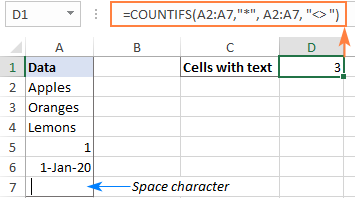If your target range contains any formula-driven data, some of the formulas may result in an empty string (""). To ignore cells with empty strings too, replace "*" with "*?*" in the criteria1 argument:

`=COUNTIFS(A2:A9,"*?*", A2:A9, "<> ")`

A question mark surrounded by asterisks indicates that there should be at least one text character in the cell. Since an empty string has no characters in it, it does not meet the criteria and is not counted. Blank cells that begin with an apostrophe (') are not counted either.

In the screenshot below, there is a space in A7, an apostrophe in A8 and an empty string (="") in A9. Our formula leaves out all those cells and returns a text-cells count of 3: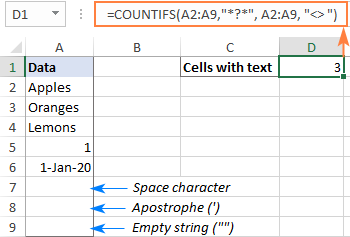## How to count cells with certain text in Excel

To get the number of cells that contain certain text or character, you simply supply that text in the criteria argument of the COUNTIF function. The below examples explain the nuances.

To match the sample text exactly, enter the full text enclosed in quotation marks:

COUNTIF(range, "text")

To count cells with partial match, place the text between two asterisks, which represent any number of characters before and after the text:

COUNTIF(range, "*text*")

For example, to find how many cells in the range A2:A7 contain exactly the word "bananas", use this formula:

`=COUNTIF(A2:A7, "bananas")`

To count all cells that contain "bananas" as part of their contents in any position, use this one:

`=COUNTIF(A2:A7, "*bananas*")`

To make the formula more user-friendly, you can place the criteria in a predefined cell, say D2, and put the cell reference in the second argument:

`=COUNTIF(A2:A7, D2)`

Depending on the input in D2, the formula can match the sample text fully or partially:

• For full match, type the whole word or phrase as it appears in the source table, e.g. Bananas.
• For partial match, type the sample text surrounded by the wildcard characters, like *Bananas*.

As the formula is case-insensitive, you may not bother about the letter case, meaning that *bananas* will do as well.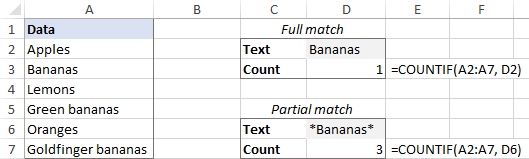Alternatively, to count cells with partial match, concatenate the cell reference and wildcard characters like:

`=COUNTIF(A2:A7, "*"&D2&"*")`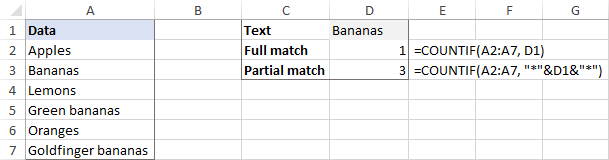## How to count filtered cells with text in Excel

When using Excel filter to display only the data relevant at a given moment, you may sometimes need to count visible cells with text. Regrettably, there is no one-click solution for this task, but the below example will comfortably walk you through the steps.

Supposing, you have a table like shown in the image below. Some entries were pulled from a larger database using formulas, and various errors occurred along the way. You are looking to find the total number of items in column A. With all the rows visible, the COUNTIF formula that we've used for counting cells with text works a treat:

`=COUNTIF(A2:A10, "*")`

And now, you narrow down the list by some criteria, say filter out the items with quantity greater than 10. The question is – how many items remained?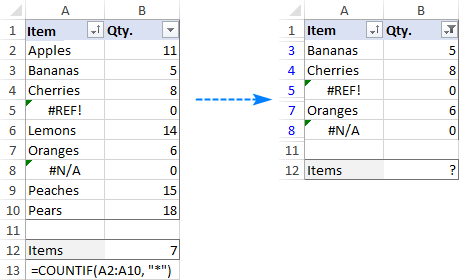To count filtered cells with text, this is what you need to do:

1. In your source table, make all the rows visible. For this, clear all filters and unhide hidden rows.
2. Add a helper column with the SUBTOTAL formula that indicates if a row is filtered or not.
To handle filtered cells, use 3 for the function_num argument:

`=SUBTOTAL(3, A2)`

To identify all hidden cells, filtered out and hidden manually, put 103 in function_num:

`=SUBTOTAL(103, A2)`

In this example, we want to count only visible cells with text regardless of how other cells were hidden, so we enter the second formula in A2 and copy it down to A10.

For visible cells, the formula returns 1. As soon as you filter out or manually hide some rows, the formula will return 0 for them. (You won't see those zeros because they are returned for hidden rows. To make sure it works this way, just copy the contents of a hidden cell with the Subtotal formula to any visible say, say =D2, assuming row 2 is hidden.)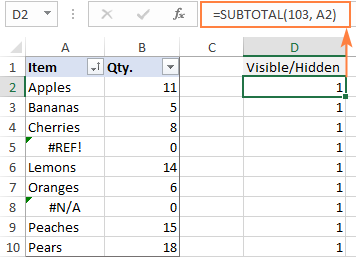3. Use the COUNTIFS function with two different criteria_range/criteria pairs to count visible cells with text:
• Criteria1 - searches for cells with any text ("*") in the range A2:A10.
• Criteria2 - searches for 1 in the range D2:D10 to detect visible cells.

`=COUNTIFS(A2:A10, "*", D2:D10, 1)`

Now, you can filter the data the way you want, and the formula will tell you how many filtered cells in column A contain text (3 in our case):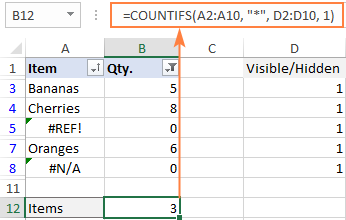If you'd rather not insert an additional column in your worksheet, then you will need a longer formula to accomplish the task. Just choose the one you like better:

`=SUMPRODUCT(SUBTOTAL(103, INDIRECT("A"&ROW(A2:A10))), --(ISTEXT(A2:A10)))`

`=SUMPRODUCT(SUBTOTAL(103, OFFSET(A2:A10, ROW(A2:A10) - MIN(ROW(A2:A10)),,1)), -- (ISTEXT(A2:A10)))`

The multiplication operator will work as well:

`=SUMPRODUCT(SUBTOTAL(103, INDIRECT("A"&ROW(A2:A10))) * (ISTEXT(A2:A10)))`

`=SUMPRODUCT(SUBTOTAL(103, OFFSET(A2:A10, ROW(A2:A10)-MIN(ROW(A2:A10)),,1)) * (ISTEXT(A2:A10)))`

Which formula to use is a matter of your personal preference - the result will be the same in any case: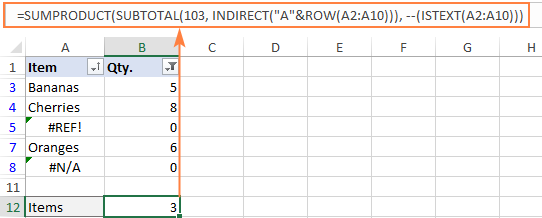### How these formulas work

The first formula employs the INDIRECT function to "feed" the individual references of all cells in the specified range to SUBTOTAL. The second formula uses a combination of the OFFSET, ROW and MIN functions for the same purpose.

The SUBTOTAL function returns an array of 1's and 0's where ones represent visible cells and zeros match hidden cells (like the helper column above).

The ISTEXT function checks each cell in A2:A10 and returns TRUE if a cell contains text, FALSE otherwise. The double unary operator (--) coerces the TRUE and FALSE values into 1's and 0's. At this point, the formula looks as follows:

`=SUMPRODUCT({0;1;1;1;0;1;1;0;0}, {1;1;1;0;1;1;0;1;1})`

The SUMPRODUCT function first multiplies the elements of both arrays in the same positions and then sums the resulting array.

As multiplying by zero gives zero, only the cells represented by 1 in both arrays have 1 in the final array.

`=SUMPRODUCT({0;1;1;0;0;1;0;0;0})`

And the number of 1's in the above array is the number of visible cells that contain text.

That's how to how to count cells with text in Excel. I thank you for reading and hope to see you on our blog next week!

## You may also be interested in

1. My example data:
4B
4A*
4A*
4A
3A* 1B
3A* 1B
3A* 1b
3A* 1A
3A* 1a
3A* 1A
3A* 1A
3A 1D
3A 1d
2C 1D 1d
2C 1D

I need to count this data. 4A* should have 2 and 4A should have 1, but they will count and combine all become 3. I also have 3A 1D and 3A 1d which each should count as 1. Can you help me to count the exact data?

2. Thank you so much for he countifs explaination it really helped me out for a work project.

3. =COUNTIF(E6:AV6, ">0")

WORKS TO COUNT THE CELLS WITH NUMBERS ONLY

4. As i am doing payment follow with customers.
I have made format for 4 times payment follow up with customers.
For example:
Column A - Date
Column B - Paid/ not paid
As per 4 times follow up schedule it repeats upto column H
Now, i want to count total number of paid in specific dates.

Help me with formula to do so.

Will be thankful.

Regards,
Nimesh Palikhel

5. Hello
I am trying to use same formula to count the specific text , if put number 1 ,2 ,3 in cell its shows the correct result . but when i am putting time i.e. 5:45 to 1:30 its show incorrect result . Kindly help.

=SUMIF(\$D\$6:\$D\$14,"OPEN",F6:F14) + SUMIF(F6:F14,"D/O",F6:F14)

6. Hi,

im using this formula to calculate the amount of quotes i do with different reasons.

how do i get it to only count them for a certain month i.e. april?

• Hello!
Use the COUNTIFS function to count with multiple conditions. An example of a condition with dates can be found in this guide.
For example:

=COUNTIFS(G1:G129,”Quoted”,D1:D129," > = 4/1/2022",D1:D129," < 5/1/2022")

7. Thank you, thank you, thank you. I had a column of cells with names, like an attendance register, with several blank cells. I wanted to count the number of cells with names but not the empty cells. Unfortunately Excel counts spaces as text, so using COUNTA wouldn't work.
The formula suggested to not count cells with spaces, =COUNTIFS(A2:A7,"*?*",A2:A7,""""), worked. Then, the explanation on how the "*?*" operator worked made me wonder if I could use that with a COUNTIF statement and that seemed work also. =COUNTIF(A2:A7,"*?*")

8. I want to Count Column A item according to Column B ... please help me how to count column A item into column B

9. Hi
I have a table with sales data for the full year, I want to Count how many Orders are Won or Lost per salesperson each month so it can auto-populate pie chart data, I "Think" I need a count if but never used the formula before. Happy to share my table and explain further if anybody can help but basically:
Column A = Salesperson
Column B = Date
Column C = Feedback (Ordered or Lost)

I want to auto-populate another table that shows how many orders a certain salesperson has won in January then February etc...

• Hello!
You can use a pivot table to count the number of orders per month. You can also use a formula. For example, customer orders for February -

=SUMPRODUCT(--(A1:A10="Salesperson"),--(MONTH(B1:B10)=2),--(C1:C10="Ordered"))

I recommend reading this guide: Conditionally count / sum / average cells.

10. Hello,

We have a large spreadsheet which tracks the hiring process for a large organization with multiple Bureaus and Departments.

I am trying to determine the formula for the following scenarios so I can create a "dashboard" summary on a different worksheet:

I need to determine the number of Job Titles per Bureau. The info resides in 2 columns. Column D lists the Bureau Name (there are 8 Bureaus listed. An example of a Bureau name is BAM) and Column G lists the Job Titles (currently there are 123 different titles in the data). I need to determine the unique job titles per Bureau.

I also need to do the same thing for Departments. There are 24 different departments and they are listed in Column E.

Please help! I've tried different combinations of COUNTIFS, COUNTA, IFERROR but I haven't landed on the right solution.

I also need to determine the number of Job Titles per Bureau

• Hi,

I tried that already and it didn't work. I need to count the unique Job Titles in Column G. As stated above, there are currently 123 unique job titles in a column of about 400 entries. The example in the link outlines looking for specific information (Basketball and <18) so the formula in the link doesn't work.

• Nevermind! I figured it out.

The winning formula was =IFERROR(ROWS(UNIQUE(FILTER('Hiring Process'!G4:G500,'Hiring Process'!D4:D500=D2))), 0)

• Hi!
Did you read the article I recommended?
You wrote: "I need to determine the unique job titles per Bureau". The formula, from paragraph 5, is suitable for this case. Now you write something else: "I need to count the unique Job Titles in Column G". The formula for this is in the first paragraph.

• Hi,

I used this formula: =IFERROR(ROWS(UNIQUE(FILTER('Hiring Process'!G4:G500,'Hiring Process'!D4:D500=D2))), 0) but the result is 1 higher than what it should be. I cannot figure out what should change. Can someone help? Maybe someone can jump on a Zoom/Teams call?

• So after using the formula above, we aren't getting accurate results. The results are 1 higher than what they should be and I'm not sure why. Can someone help?

• Hi!
Without seeing your data it is difficult to give you any advice.
I can assume that one of the values in your data has extra space or some other character that you don't see. Check it out.

• We think we've figured out the problem, but don't know exactly how to solve it in the formula.

We believe the formula is counting all blank cells as one unique value, hence why it the results are higher. We found the "" solution, but do not know where to put it within the formula we are using. We've tried a number of places and nothing is coming up with the right answer.

How can we filter out blanks while also filtering on multiple criteria? Any advice or assistance would be appreciated!

THANK YOU!

11. Respected Sir,
What is the formula for counting text of different cell which is not in a range. for Example how to calculate text of A1+C1+F1

• Hi!
What does the “calculate text” phrase mean?

• text mean any word in the cell. It may be 'teacher' or 'monkey' etc

• Hi!
Here is the article that may be helpful to you: How to count words in Excel.
If this is not what you wanted, please describe the problem in more detail.

12. Hi, Thank you so much for this!!

One question though. I have a row of cells that shows this [Logo: Diamond, Size: Medium Devlievery: Pick up)

I am using the formula you explained as such:

=COUNTIF(A2:A7, "*Diamond*")

to calculate the number of times "Diamond logo appears in the row. But I also want to have a cell return me the total amount of "Medium size t shirts with Diamond logo"

I tried this:

=COUNTIF(A2:A7, "*Diamond*" + "*Medium*")

Did not work obviously. However, what formula should I use to do so?

13. Hi,

how do I convert the below to be able to use using a filter:

I will be filtering from month to month in yearly data to grab the above.

Thanks all!

• Hi!
Sorry, it's not quite clear what you are trying to achieve. Explain in more detail.

14. Hello,
I have a spreadsheet that has a list of categories using roman numerals I to VI. The spreadsheet has over 6000 rows (each row is a assigned to a hospital room) and I filter it for most applications. I want to be able to count specific categories after filtered. Once it is filter and I can count manually 20 Cat I's and 13 Cat VI's but my current formula counts all filtered and unfiltered. What formula would count these characters separately after being filtered and give a total of each cell in a column that has these specific characters? at the bottom of the page. The bottom of the column would look like this and not count unfiltered data.
Cat I = 22
Cat II = 23
Cat III= 15
Cat IV= 32
Etc.... I hope this makes since.
V/r
Ron Lambert

• Hello!
Based on your description, it is hard to completely understand your task. However, I’ll try to guess and offer you the following formula:

=COUNTIF(A2:A1000, "*Cat I*")

If this is not what you wanted, please describe the problem in more detail.

15. Hi,
Good day!

If(‘May2021’!C:C,”=Tony”, countif(‘May2021’!D:Z,”=Present”)

Thank you so much

16. HI,

Good day!

can you help me if this is possible to do in excel, I want to count the number of cells containing the specific value that is within a specific row or column that is in between a specific value in a cell.

i.e. COUNT THE CELLS IN A ROW BETWEEN "JAN"

A B C D E F G H I
1 TOTAL COUNT
2 JAN FEB MAR OCT SEPT MAR JAN AUG 5
3 FEB JAN DEC FEB JAN APR MAR OCT 2
4

I.E. COUNT THE NUMBER OF CELLS HAVING "P" BETWEEN "O".

A B C D E F G H I
1 TOTAL COUNT
2 O P P P P P O P 5
3 P O P P O P P P 2
4

17. I have a spreadsheet that has a column for each month (12 columns) and a row for each day (31 rows), I have put in each column and row a letter to track type of days off, I want to total the types of days off on a separate spreadsheet. Is there a formula that will do this for me?

18. Hi,
I was wondering if there is a way to count the total number of entries for one certain type of information, I have a spreadsheet with over 500 entries but I need to know the total entries for one particular key word

hope this makes sense

thank you

• Hello!
Have you tried the ways described in this blog post? If they don’t work for you, then please describe your task in detail, I’ll try to suggest a solution.

19. Hallo,

I have a table with 10 columns and 240 rows. Every cell contains a product in text format. Is there any formula to count the total number of every product in the whole table without putting any criteria for searching any product. Because the products are more than 100.

Thanks

• Hello!
Give an example of the source data and the expected result. Do you want to get a list of all products along with the quantity of each product? For this I recommend using a pivot table. If this is not what you wanted, please explain the problem in more detail.

• Hello,
By using a pivot table I can count column by column. I want to count the numbers from all columns automatically without specifying a criteria for each product. Here is the example:

Database Final table

A B D F G A number
B C A I H B number
C A B L A C number
E F G C A D number
K I H E F E number
L A M A I F number
…. …. …. …. …. … number
…. …. …. …. …. … number
…. …. …. …. …. … number
…. …. …. …. …. … number
…. …. …. …. …. … number
…. …. …. …. …. … number
…. …. …. …. …. … number
…. …. …. …. …. … number
…. …. …. …. …. … number

• It's not visible. Now I think it's better.

Database

A B D F G
B C A I H
C A B L A
E F G C A
K I H E F
L A M A I
…. …. …. …. ….
…. …. …. …. ….
…. …. …. …. ….
…. …. …. …. ….
…. …. …. …. ….
…. …. …. …. ….
…. …. …. …. ….
…. …. …. …. ….
…. …. …. …. ….

Final table

A number
B number
C number
D number
E number
F number
… number
… number
… number

• Hello!
Use this formula to extract unique values from a range.
Assuming your values ​​are in the range A2: C9, enter the following formula in cell E2:

=INDIRECT(TEXT(MIN(IF((\$A\$2:\$C\$9<>"") * (COUNTIF(\$E\$1:E1,\$A\$2:\$C\$9)=0), ROW(\$2:\$9)*100+COLUMN(\$A:\$C),7^8)),"R0C00"),)&""

In the above formula, A2:C9 indicates the range of cells from which you want to extract unique values. E1 is the first cell in the column where you want to place the result. \$2:\$9 means strings containing cells you want to use. \$A:\$C points to the columns containing the cells you want to use. Please change them to your own.
Press Shift + Ctrl + Enter and then drag the fill handle to extract unique values ​​until blank cells appear.

To count the number of each unique value in a range use the formula

=SUM(--(TEXT(\$A\$2:\$C\$9,"General")=TEXT(E2,"General")))

I entered the first formula exactly as you explained.
It gives me an error in the part "E1,\$A\$2"

=INDIRECT(TEXT(MIN(IF((\$A\$2:\$C\$9"") * (COUNTIF(\$E\$1:E1,\$A\$2:\$C\$9)=0), ROW(\$2:\$9)*100+COLUMN(\$A:\$C),7^8)),"R0C00"),)&""

Rgds
Ivan

• Hi,
What separators do you use in your formulas? I guess it's a semicolon. Consider your regional settings.

• Hi,

yes, the separator is a semicolon. Should I change it?

May I send you my table via e-mail in order that you put the formulas. Which e-mail?

Thanks
Ivan

• Hi,
Change the commas in the formula to a semicolon.

• Hi,
I changed the commas in the formula to a semicolon.

Now the formula shows me an error in the part \$C\$9""

Rgds
Ivan

• Hi!
Copy the formula carefully. Check all signs. I repeat it one more time

=INDIRECT(TEXT(MIN(IF((\$A\$2:\$C\$9 < > "")*(COUNTIF(\$E\$1:E1,\$A\$2:\$C\$9)=0), ROW(\$2:\$9)*100+COLUMN(\$A:\$C),7^8)),"R0C00"),)&""

\$C\$9"" is incorrect. Need to \$C\$9 < > ""
Remember to press Shift + Ctrl + Enter. This is an array formula.

• Hi,

I copied your new formula in the cell E2. When pressing Shift + Ctrl + Enter it appears a message that says me that \$C\$9 is incorrect and suggests me a formula with \$C\$9 < only. Even with this element the cell E2 remains blank.

Rgds
Ivan

• Hi,
The part of the formula \$C\$9 "" is not accepted. It suggests me a formula with \$C\$9 < "" only. Even with this element the cell E2 remains blank.

Ivan

• The symbols higher and lower do not appear in the post. I don't know why. It suggests me a formula only with the symbol lower.

• \$C\$9

• doesn't appear correctly in the post here

• Actually above formula works but u have to cut space between in =INDIRECT(TEXT(MIN(IF((\$A\$2:\$C\$9 ""). Beyond that you have to put this function into E2 cell (not E1) and then stretch to bottom cells until unique values will stop appearing. It was achieved by trials and errors, so I cant explain why it so, but it works.

20. I may have missed this in your above info, but how do I count a row of 'text' that contains both dates and text? I am trying to get a subtotal of tasks undertaken by a list of clients.

• Hello!
If I understand your task correctly, You cannot perform mathematical operations on text. Therefore, you must first select the dates or numbers that you need from the text. The information you provided is insufficient for a more accurate answer.

21. Is there any why to use SUBTOTAL with COUNTIF?
I would like to count cells with text from a filtered list of text.
I know how to do this with adding numbers but not counting text.

Thank you,
Ray

22. A lot of useful information,

Thank you,
Ray

23. Hello
let say i have 4 sheet name (A,B,C & D)
it is actually days shift report , mean same format but different fault log of equipments.
let say there are 4 equipments ( Eq-1,Eq-2,Eq-3 & Eq-4) in one colum and second colum has its different fault types like( brake fault, hoist fault, run fault etc..)
same fault log could be in all sheets (A,B,C & D).
now on one sheet say summary i want to count Eq-1 brake fault what how many time it occurs total in all 4 sheets.
i hope i have explained details about my requirement.

Thanks,
asif

24. Hello,
From the example of =COUNTIF(A2:A7, "bananas")
What formula would I use to have that same row count BANANAS and APPLES at the same time.

• Hi Ana,

If my understanding is correct the task is to count cells in A2:A7 that contain either "bananas" or "apples".

The easiest solution is to add up 2 COUNTIF functions:

=COUNTIF(A2:A7, "bananas") + COUNTIF(A2:A7, "apples")

Or you can use a SUM COUNTIF formula with an array constant:

=SUM(COUNTIF(A2:A7, {"apples","bananas"}))

For full details, please see COUNTIF and COUNTIFS with multiple OR conditions.

25. Hey there
I have a sheet that in column B has the location (ex, Cali/ Texas) and the column D - I tally all the ones from Cali aka California and Column E - I tally all the ones from Texas
Ex:
Col B Col D Col E
Cali 1
Texas 1
- My question is, is there a way to have column D auto generate a "1" when column B states Cali and vise versa for column E auto generate a "1" when column B states Texas.

26. Hi, I have a sheet which I'm using to calculate how many sales are from which or 10 companies. Column B has customers names, column aa has the main company name, column aj is closed (not sold) and column ao has the dates something was sold. I have a table on a separate tab with this formula: =COUNTIF(Main!AA:AA,A2) main is the tab name, AA the column and A2 is the cell with the company name I'd like to count. My question is, what formula what I use if I wanted to count these names only if sold (based on any text in column ao), closed (based on any text in column aj) and still outstanding based on no text in ao or aj. Hopefully that makes sense! Thanks

• ps. I should point out that I'm counting the number of cells, not the value within those cells as they all contain text or dates. But if it is also possible to count the value of columns AL, AM, and AN based on the company name in column AA, then that would be amazing.

27. I have a column with various names and another column with various statuses attached to those names. I want to distinguish specific statuses attached to these names.
E. G.
Column A and B will look like this:
Mr A. Status complete
Mr A. Status outstanding
Mr A status paid
MR B status outstanding
MR B status complete

I need to which of these rows per name contain a combination of statuses complete and paid.
In example above Mr A must be shown to have a status in 2 of the rows and Mr B will not show up anything.

28. HOW TO USE A FUNCTION THAT WILL CALCULATE THE TOTAL NUMBEROF PRODUCT THAT ARE IN TEXT

29. I have attendance sheet to prepare so I want to count number of p in the same row from 1st September to 30th September. how do I do it?

30. Hi Team

I have been battling for ages to get this formula right,

=COUNTIF('Day 1:Day 31'!J8:J25,"CORGI")

I have a 12 mth workbook with and column J8:j25 that has names in text ie Corgi,Sandra,Stuart,House etc.

i can use the countif to find the individual totals on a single work sheet but that takes up a lot of time and space , i am looking the formula to use on a summary sheet and this is the best thing i have come up with but does not work, for some reason the J8:j25 just relates to the summary sheet i iam working on.

could you please shed some light on where i am going wrong?

Many thanks
Robert

31. Hello, I'm trying to get a row to tally if a range has either P,S, or 2 within the range. Is there a way to accomplish this?

• Hello!
I’m sorry but your task is not entirely clear to me. For me to be able to help you better, please describe your task in more detail. Please specify what you were trying to find, what formula you used and what problem or error occurred. Give an example of the source data and the expected result.
It’ll help me understand it better and find a solution for you. Thank you.

32. Im looking for a formula to give me only the number of managers I have working projects. Each manager has multiple projects they work on. Manager names are in column A. Projects in B. I only want to know the total managers I have, not the projects I have. For instance, John's name is listed 8 times, Mike's name is listed 6 and Jill's name is listed 7 times. The answer I want is 3.

33. Is there a formula that will count all cells containing text within a specific range except cells that contain certain a certain word or character (in my case, I need a count that includes everything in the spreadsheet except blank cells and cells that contain "X"). Thank you!

34. hello sir,
i have a 03 criterias (higher,lower and standard)in a column which ranges from (8-34200 rows)i also have date column now my problem is whenever i makes a filter in date for example if i want to check how many higher ,lower cases are occured on particular date

• Hello!
I’m sorry but your task is not entirely clear to me. For me to be able to help you better, please describe your task in more detail. Please specify what you were trying to find, what formula you used and what problem or error occurred. Give an example of the source data and the expected result.
It’ll help me understand it better and find a solution for you. Thank you.

• Hello!
If I understand your problem correctly, you need to use the COUNTIFS function to count. Read more here.

35. hi. Need help.
I have a row of numbers and text "IN" (in different cells).
What formula do I use to count the total if any of the cells in the row have numbers and IN.

• Hello!